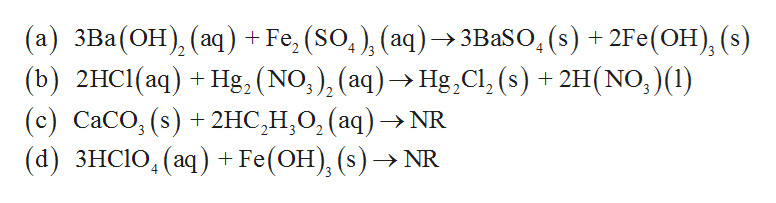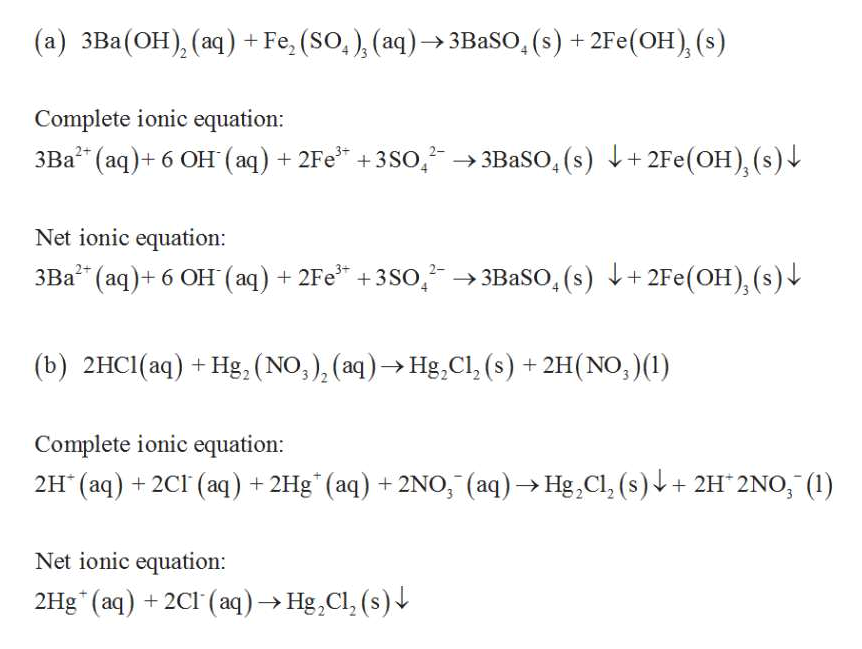# Predict products and balance the following reactions. If no reaction takes place, write NR. Be sure to include phases. Find molecular, total-ionic, and net ionic equations for the following:a. Ba(OH)2 (aq) + Fe2(SO4)3 (aq) ->b. HCl (aq) + Hg2(NO3)2 (aq) ->c. CaCO3 + HC2H3O2 (aq) ->d. HClO4 (aq) + Fe(OH)3 (s) ->

Question
200 views

Predict products and balance the following reactions. If no reaction takes place, write NR. Be sure to include phases. Find molecular, total-ionic, and net ionic equations for the following:

a. Ba(OH)2 (aq) + Fe2(SO4)3 (aq) ->

b. HCl (aq) + Hg2(NO3)2 (aq) ->

c. CaCO3 + HC2H3O2 (aq) ->

d. HClO4 (aq) + Fe(OH)3 (s) ->

check_circle

star
star
star
star
star
1 Rating
Step 1

The products for given reaction is represented by using the following molecular balanced chemical equation.help_outlineImage Transcriptionclose(а) зВа(ОН), (аq) + Fe, (SO,), (aq) ЗВaSO, (s) + 2Fe(ОН), (:) (b) 2HCI(аq) + Нg, (NO,), (aq) -> Нg,CIl, (с) СаСО, (s) + 2HC,Н,О, (аq) >NR (а) ЗHСIO, (аq) + Fe(ОH), (s) ->1 4 (s) + 2H(NO, )(1) fullscreen
Step 2

The complete ionic and net ionic ...help_outlineImage Transcriptionclose(a) 3Ba(OH), (aq) +Fe, (So,) (aq)3BaSO, (s) + 2Fe(OH), (s) Complete ionic equation: 3BaSO, (s) +2Fe(OH), (s) 3Ba2 (aq)6 OH (aq) 2Fe +3So, 2- Net ionic equation 3Ba (aq)6 OH (aq) 2Fe +3SO,2 3BaSo, (s) +2Fe(OH), (s) (b) 2HC1(aq) +Hg, (NO,), (aq) Hg,Cl, (s)+2H(NO, )() Complete ionic equation: 2H (aq)+2CI (aq) +2Hg (aq)+2NO, (aq)Hg,Cl, (s)+ 2H 2NO, () Net ionic equation: 2Hg(aq) 2C1 (aq)Hg,Cl, (s) fullscreen

### Want to see the full answer?

See Solution

#### Want to see this answer and more?

Solutions are written by subject experts who are available 24/7. Questions are typically answered within 1 hour.*

See Solution
*Response times may vary by subject and question.
Tagged in
ScienceChemistry

### General Chemistry